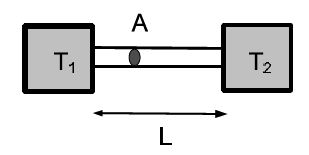Physics

# What is Temperature and Heat?

While a formal definition of temperature is outside the scope of introductory physics, “temperature is the thing that’s the same for two objects after they’ve been in contact long enough”. When the two bodies are in contact, they will exchange energy with each other. Temperature is the quantity that describes such a spontaneous flow of energy [heat] between objects at different temperatures. Energy flows from the hotter object to the colder object. Two ojects have the same temperature when there is no net flow of heat between them. The zeroth law of thermodynamics states that two bodies, each in thermal equilibrium with a third, are also in thermal equilibrium with each other.

Properties of water [H2O] are used to define the Celsius temperature scale. Liquid water and ice coexist at Tc = 0°C and liquid water and vapor coexist at TC = 100°C. The absolute temperature scale is:

T = TC + 273.15

The unit of temperature is [T] = K (Kelvin).Heat flow: Heat flow is the rate of energy transfer or Q/t. Two objects are kept at temperatures T0 and T1, respectively. The two bodies are connected by a heat conductor [e.g., a metal strip] of length L and cross-sectional area A. The flow of heat depends on the “steepness” the temperature change ΔT/L [temperature gradient]. The heat flow is proportional to the cross-sectional area A:

Q/t = k *A* (ΔT/L)

Here, k is the thermal conductivity with unit [k] = W/(Km), and must, in general, be measured experimentally. Good heat conductors are also good conductors of electricity. Thus metals are good heat conductors, whereas insulators [wood and (most) plastics] are bad conductors. Humans are sensitive to heat flow, and not the temperature. After a cold winter night, the wood on the porch ‘feels’ warmer than the metal railing, although they are at the same temperature. The different “feel’ is due to the difference in thermal conductivities of the two materials [greater for metal than for wood].

Heat can also be transferred through (blackbody) radiation,

where A is the surface area, e is the emissivity [ e < 1 ] and σ is the Stefan-Boltzmann constant σ = 5.67 x 10-8 W/(m2 • K4).